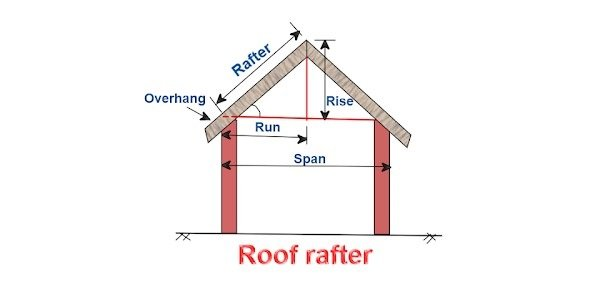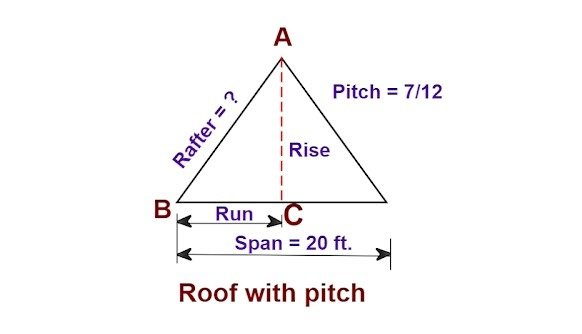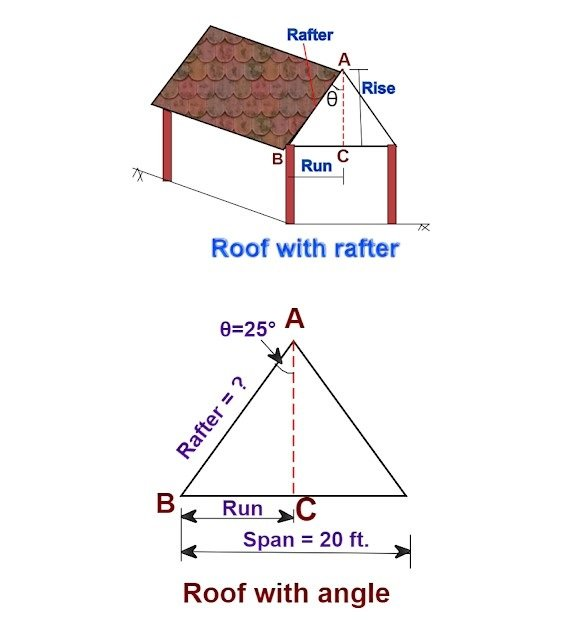Breaking News
Home / Civil Engineering / How To Calculate The Length Of Roof Rafters

# How To Calculate The Length Of Roof Rafters

## How To Calculate The Length Of Roof Rafters

Let us calculate the length of the roof rafter as shown in the below drawing.The length of the roof rafters is calculated in three different methods based on the available data. Let us go through, all of them as explained below.

### 1. Roof rafter with pitch:Given data:

Span = 20 ft.

Pitch = 7 /12

As you know,

Run = [span ÷ 2]

= [ 20 ft. ÷ 2]

### Here,

Pitch = [rise / run.]

i.e.   ( 7 / 12 ) = [ rise / 10 ft.]

By cross multiplication,

Rise = [ (7/12 ) × 10 ft.]

### = 5.833 ft.

By Pythagoras theorem,

AB2= AC2 + BC2

i.e. rafter2= rise2 + run2

Rafter = √ rise2 + run2

Rafter = √ (5.833)2 + (10)2

= √ 34.027 + 100

= √ 134.027

1. ### Roof rafter with an angle & span:Span = 20 ft.

Angle θ = 25°

As you know,

Run = [span ÷ 2]

= [ 20 ft. ÷ 2]

### = 10 ft.

By trigonometry,

sinθ = opposite / hypotenuse

In the above triangle,

Side AB = rafter = hypotenuse

Side BC = run = opposite.

### So,

Sin25° = [ 10 ft. ÷ hypotenuse]

0.4226 = [ 10 ft. ÷ hypotenuse]

By cross multiplying,

Hypotenuse = [10 ft. ÷ 0.4226 ]

### Given data :

Rise = 8 ft.

Angle θ = 25°

By trigonometry,

In the above triangle,

Side AB = rafter = hypotenuse

Side AC = rise = adjacent.

So,

Cos25° = [ 8 ft. ÷ hypotenuse]

0.906 = [ 8 ft. ÷ hypotenuse]

By cross multiplying,

Hypotenuse = [8 ft. ÷ 0.906 ]

## . Land Surveying & Architects

### THANKS.